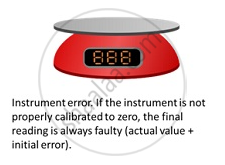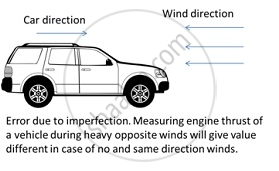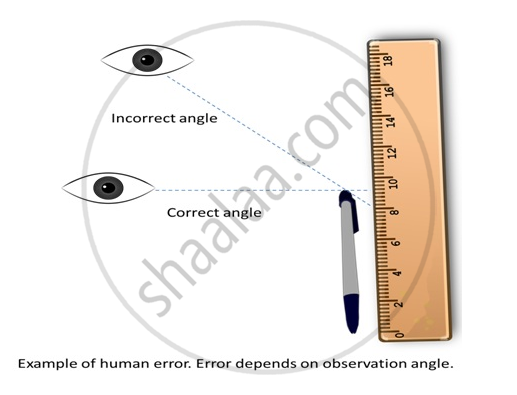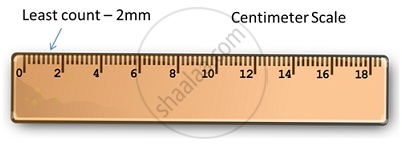# Accuracy, Precision and Least Count of Measuring Instruments

#### description

• Accuracy of measuring instruments
• Precision of measuring instruments
• Least count for various instruments
• Zero error: Negative and Positive zero error

## Accuracy, Precision of Instruments and Errors in Measurements

Measurement is the foundation of all experimental science and technology. The result of every measurement by any measuring instrument contains some uncertainty. This uncertainty is called error. Every calculated quantity, which is based on measured values, also has an error.

Accuracy: The accuracy of a measurement is a measure of how close the measured value is to the true value of the quantity.

Precision: Precision tells us to what resolution or limit the quantity is measured.

For example:- If the true value of a certain length is 3.678 cm and two instruments with different resolutions, up to 1 (less precise) and 2 (more precise) decimal places respectively, are used. And if first measures the length as 3.5 and the second as 3.38 then the first has more accuracy but less precision while the second has less accuracy and more precision.

Thus every measurement is approximate due to errors in measurement.

In general, the errors in measurement can be broadly classified as follows:-
(a) Systematic errors
(b) Random errors.

Systematic Error
The systematic errors are those errors that tend to be in one direction, either positive or negative. Errors due to buoyancy in weighting and radiation loss in calorimetry are systematic errors. They can eliminated by manipulation. Some of the sources of systematic errors are

1. Instrument errors: These arise from imperfect design or calibration error in the instrument. Worn off scale, zero error in a weighing scale are some examples of instrument errors.2. Imperfections in experimental techniques: If the technique is not accurate (for example measuring temperature of human body by placing thermometer under armpit resulting in lower temperature than actual) and due to the external conditions like temperature, wind, humidity, these kinds of errors occur.3. Personal errors: Errors occurring due to human carelessness, lack of proper setting, taking down incorrect reading are called personal errorsThese errors can be removed by:

• Taking proper instrument and calibrating it properly.

Experimenting under proper atmospheric conditions and techniques.

Random errors

• Errors which occur at random with respect to sign and size are called Random errors.
• These occur due to unpredictable fluctuations in experimental conditions like temperature, voltage supply, mechanical vibrations, personal errors etc.

Least Count Error
Smallest value that can be measured by the measuring instrument is called its least count. Least count error is the error associated with the resolution or the least count of the instrument.

• Least count errors can be minimized by using instruments of higher precision/resolution and improving experimental techniques (taking several readings of a measurement and then taking a mean).

• Least count error belongs to the category of random errors but within a limited size; it occurs with both systematic and random errors.

For example, a vernier callipers has the least count as 0.01cm; a spherometer may have a least count of 0.001 cm.Absolute Error, Relative Error and Percentage Error

Absolute Error:-
If a1,a2,a3,....,an be the measured values of a quantity in several measurements, then their mean is considered to be the true values of that quantity i.e.,
true value a0 = amean = [a_1 + a_2 + a_3 + ... +a_n ]/[n]

The magnitude of the difference between the true value of the quantity and the individual measurements value is the absolute error of that measurement. Hence, absolute errors in measured values are:
Δa_1 = a_0 - a_1, Δa_2 = a_0 - a_2, Δa_3 = a_0 - a_3,  ..........Δa_n = a_0 - a_n

The arithmetic mean(i.e., the mean of the magnitudes) of all the absolute error is known as mean absolute error.
Δamean = [|Δa_1| + |Δa_2| + |Δa_3| + ......+ |Δa_n|]/[n]

Relative Error:-
The ratio between mean absolute error and the mean values is called relative error

Relative error = "Mean  absolute error"/"Mean  value"

= (triangle "a"_"mean")/("a"_"mean") = (triangle "a"_"mean")/("a"_"0")

Percentage Error:-
Percentage Error is the expression of the relative error in percentage.
Percentage Error = (triangle "a"_"mean")/("a"_"mean")  × 100%

Combination of errors
If a quantity depends on two or more other quantities, the combination of errors in the two quantities helps to determine and predict the errors in the resultant quantity. There are several procedures for this.

Suppose two quantities A and B have values as A ± ΔA and B ± ΔB. Z is the result and ΔZ is the error due to combination of A and B.

 Criteria Sum or Difference Product Raised to Power Resultant value Z Z=A+-B Z=AB Z= A^k Result with error Z+- ΔZ=(A+-ΔA)+(B+-ΔB) Z+- ΔZ=(A+-ΔA)(B+-ΔB) Z+- ΔZ=(A+-ΔA)^k Resultant error range +- ΔZ=+-ΔA+-ΔB (ΔZ)/Z=(ΔA)/A+-(ΔB)/B Maximum error ΔZ=ΔA +ΔB (ΔZ)/Z=(ΔA)/A+(ΔB)/B (ΔZ)/Z=k((ΔA)/A) Error Sum of absolute errors Sum of relative errors k times relative error

If you would like to contribute notes or other learning material, please submit them using the button below.

### Shaalaa.com

Types of Error [00:08:21]
S
0%